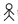YearsNumber of students19964001998535200047220026002004623
A. Prepare a pictograph of students using one symbolto represent 100 students and answer the following questions:
(a) How many symbols represent total number of students in the year 2002?
(b) How many symbols represent total number of students for the year 1998?
B. Prepare another pictograph of students using any other symbol each representing 50 students. Which pictograph do you find more informative?" ">

# Total number of students of a school in different years is shown in the following table YearsNumber of students19964001998535200047220026002004623A. Prepare a pictograph of students using one symbolto represent 100 students and answer the following questions:(a) How many symbols represent total number of students in the year 2002?(b) How many symbols represent total number of students for the year 1998?B. Prepare another pictograph of students using any other symbol each representing 50 students. Which pictograph do you find more informative?"

To do:

We have to prepare a pictograph of students using one symbolto represent 100 students and answer the given questions.

Solution:

 Year Number of students-100 students 19961998200020022004(i)  6 symbols represent total number of students in the year 2002.

(ii) 5 complete and 1 incomplete symbols represent total number of students for the year 1998.

The pictograph for the given data is as follows:

 Year Number of students               $\otimes=50$ students 1996 $\otimes$$\otimes$$\otimes$$\otimes$$\otimes$$\otimes$$\otimes$$\otimes$ 1998 $\otimes$$\otimes$$\otimes$$\otimes$$\otimes$$\otimes$$\otimes$$\otimes$$\otimes$$\otimes$ x 2000 $\otimes$$\otimes$$\otimes$$\otimes$$\otimes$$\otimes$$\otimes$$\otimes$$\otimes$x 2002 $\otimes$$\otimes$$\otimes$$\otimes$$\otimes$$\otimes$$\otimes$$\otimes$$\otimes$$\otimes$$\otimes$$\otimes$ 2004 $\otimes$$\otimes$$\otimes$$\otimes$$\otimes$$\otimes$$\otimes$$\otimes$$\otimes$$\otimes$$\otimes$$\otimes$x

Pictograph $B$ gives better approximations.

Therefore,

Pictograph $B$ is more informative.

Updated on: 10-Oct-2022

20 Views